# How-to Create a Time Data Series Step Chart in Excel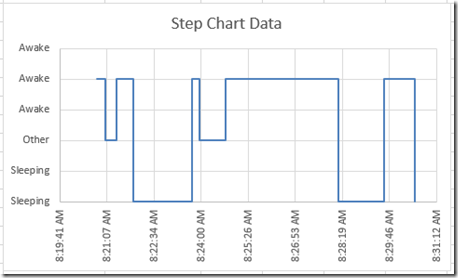In our recent Friday challenge, we were asked by Anna how can we make our popular Excel Step Chart work for data that is not date based, but time based.  The use case is a medical study on sleeping.

You can read all about it here:   Friday Challenge – Time Data Series Step Chart

Here is what the data looked like: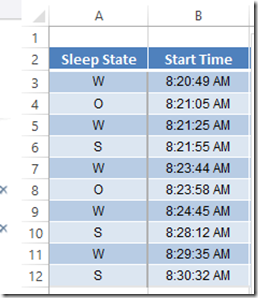Here is what the final chart will look like: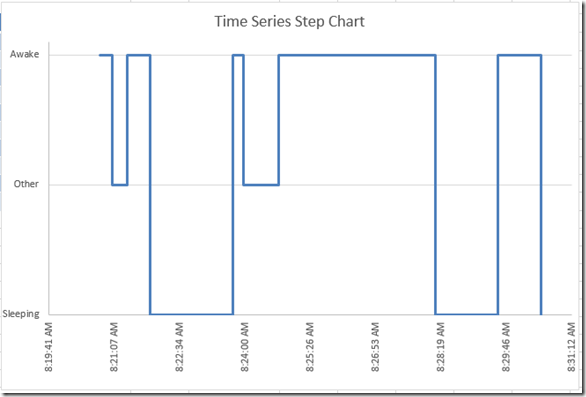The Breakdown

1) Add Column of Data to Convert Text Values to Numbers

2) Create Step Chart Horizontal Data

3) Create Step Chart Vertical Data

4) Create XY Scatter Line Chart from Chart Data

5) Change Horizontal Axis Alignment to Vertical

6) Change Number format of Vertical Axis to Custom Number Format

8) Delete Chart Junk

Step-by-Step

1) Add Column of Data to Convert Text Values to Numbers

# If you found the website and tutorials helpful, please consider donating to keep the lights on.

## Donate with PayPal here:First, we need to modify the Awake, Other and Sleeping data to numbers instead of text since Excel doesn’t know how to graph text.

Here is a similar tutorial were we fake Excel to chart text:

How-to-make-categories-for-vertical-and-horizontal-axis-in-an-excel-chart

In order to do this, we need to add a column of data that converts the W, O and S into numbers.  So in Column C, I used this formula:

C3 =IF(A3=”w”,2,IF(A3=”o”,1,0))

Essentially, this formula converts W’s to a value of 2, and converts O’s to values of 1 and all other values to 0.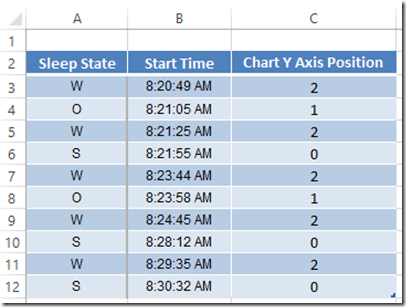We will use this data for our Vertical Axis values.

2) Create Step Chart Horizontal Data

Now we need to create the following formula in column D and copy it down:

D3   =SMALL(Table1[Start Time],TRUNC(ROW()/2,0))

What this does is copy the start time data and duplicates each value except the first value as you see here: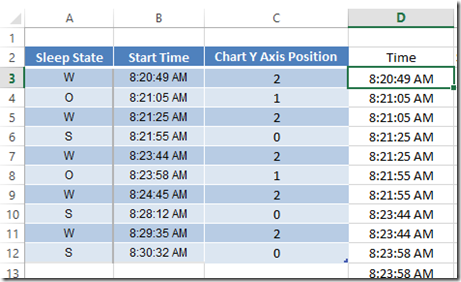Just copy it down until you see all #Num values.

3) Create Step Chart Vertical Data

Now we need to create the following formula in column E and copy it down:

E3   =IF(ISNUMBER(D2),IF(D3<>D2,E2,VLOOKUP(D3,Table2[[Start Time]:[Chart Y Axis Position]],2,FALSE)),VLOOKUP(D3,Table2[[Start Time]:[Chart Y Axis Position]],2,FALSE))

What this does is copy the chart y axis position data for the matching time and then duplicates each value one time for the next horizontal point.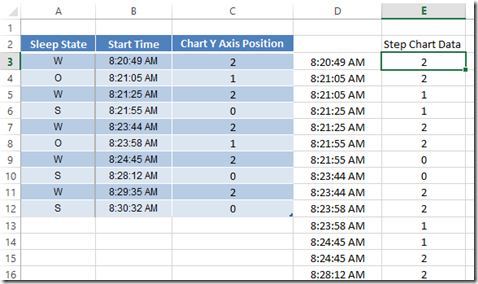4) Create XY Scatter Line Chart from Chart Data

Now that we have our data setup, we can create our chart.  Here is where we need to adjust our step chart to use a different chart type.  Normally, we can just use a line chart, but Excel doesn’t plot time data the same that it does with dates.  So a line chart won’t work.  Here is what it looks like if we chart the data as a line chart: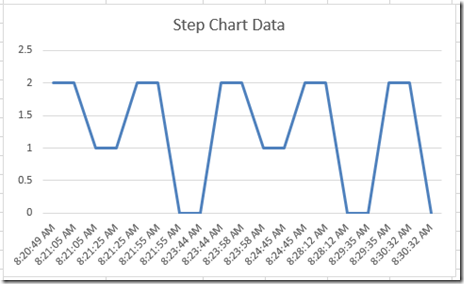So we need to pick a different type chart.  In order for Excel to treat our data correctly, we need to pick an XY Scatter with Straight Lines: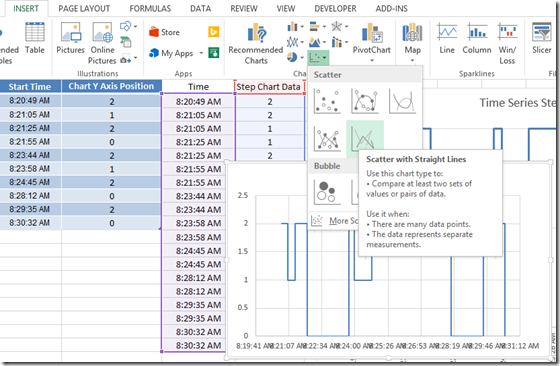If you pick that chart type, Excel will create your desired chart in a step format like you see here: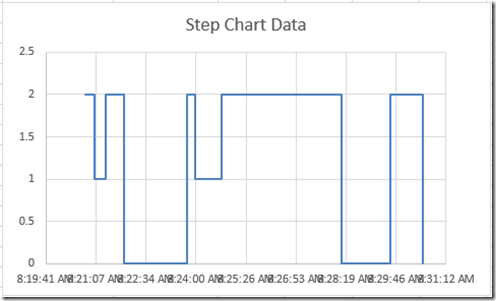5) Change Horizontal Axis Alignment to Vertical

Now there is a few things we need to fix in order to get the final Excel chart.  First, we need to make it so that we can see the horizontal axis values as they are overlapping each other.  To do this, double click on the horizontal axis time numbers.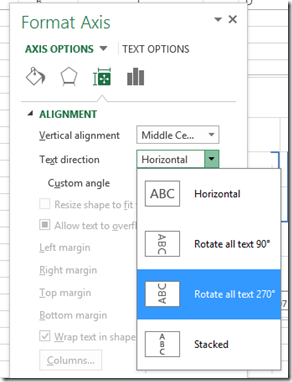Then change the alignment axis options to Rotate all text 270 degrees.  Your chart will now look like this: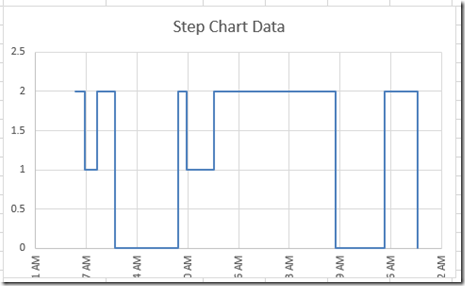Don’t worry about the numbers just yet, we will fix that in a later step.

6) Change Number format of Vertical Axis to Custom Number Format

Now our desired chart was to have text values show up in the vertical axis instead of numbers.  In order to do this, we need to trick Excel number formats.  To do this, double click on the vertical axis and add this custom number format

[>1]”Awake”;[=1]”Other”;”Sleeping”   (Note: sometimes copying this from the web to Excel, you may need to delete and replace the quotation marks)

Your chart will now look like this: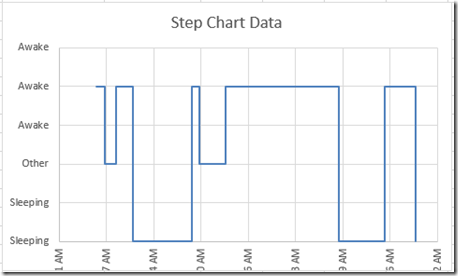Okay, lets fix the horizontal axis numbers so that we can see them.  In order to do this, we need to shrink the plot area of the chart.  First select your chart and then select the plot area as you see here: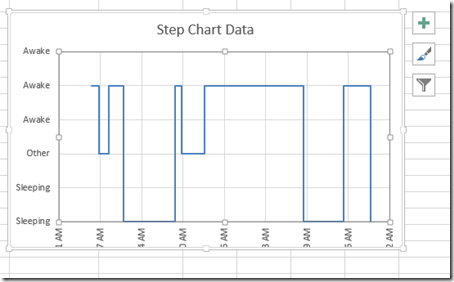Then drag and drop the bottom control box up so that the plot area gets smaller from the bottom of the chart.  Your chart will now look like this:8) Delete Chart Junk

Your chart may have vertical gridlines that you may not want.  If you don’t want them, simply click on the vertical gridlines and press your delete key.  Here is what your final chart will look like: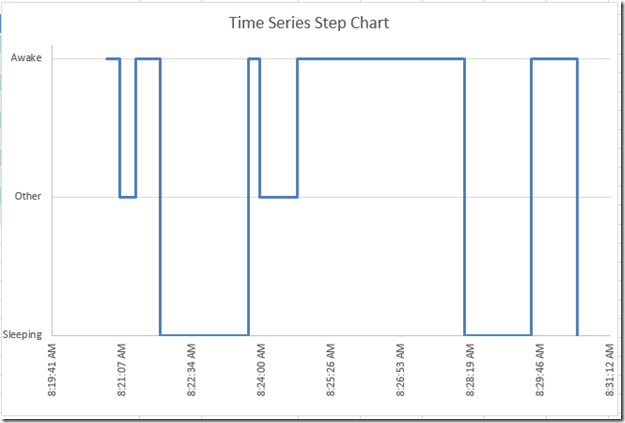Video Tutorial

Check out a video tutorial on making this chart here:

Friday Challenge Answer – Time Data Series Excel Step Chart.xlsx

If Anna’s paper is accepted, I may get an honorable mention in helping her with this graph.  How neat is that? 🙂

I believe that you can also use Excel Scatter Charts for the date step charts, but you definitely have to use them for Time Series charts.  I wonder why Excel moved away from Time Series charts?  I saw images online where this was an option in Excel 97.  If you have a copy of Excel 97, I would love to see how one of those charts converts to Excel 2013.  I only have 2003 as my oldest copy.  I would imagine that it would throw an error and not work.   Good luck with the paper submission Anna!

Steve=True

1.Vincent

Hi Steve,
I really liked this tutorial and it really helped solve my question for the most part.

However, the problem that I have now is that when I go to do the scatter plot with straight lines, the times get all messed up. The intervals are weird and it inserts times before my data time set and after.

I also, had the problem when using a line chart that it repeats the times. As in it lists all the time intervals in the data set and doesn’t skip the ones that repeat like in your example.

I am using Excel 2010. It would be great if you could help me with this. Thank you!

•SteveEqualsTrue

Hi Vincent,

Make sure your times are not Text, but Excel date/time format (I.e. it is a number). You can verify what it is by going to a new cell and putting in any calculation formula like =1+[your cell with a time].

Hope that helps.

Steve=True

•deed

thanks a lot this is great article to learn plot a time series chart setup in excel.

•SteveEqualsTrue

Thanks for the great comment deed.

• # If you found the website and tutorials helpful, please consider donating to keep the lights on.

## Donate with PayPal here:•SR

Is there a way of filling up the area below the plot?

•SteveEqualsTrue

Hi SR, yes you can but to do that you need to change the chart type to a Clustered Column chart. Then Change the Gap Width of the series to 0 (zero). However, this may give people an impression that it is a range that is not accurate in this specific example because the column stretches from sleeping to other to awake when it is really a binary or three way choice. Just my thoughts.